Properties, Synthesis and Reactions of Alkenes and Alkynes

Properties, Synthesis and Reactions of Alkenes and Alkynes

Key Terms
• sp2 hybrid orbital
• Pi (π) bond
• sp hybrid orbital
• Stereochemistry
• Alkenyl group
• van der Waals strain
• Stereoisomer
• Beta (β) elimination
• Dehydration (of alcohols)
• E1 mechanism
• E2 mechanism
• Carbocation
• Zaitsev's rule
• Dehydrohalogenation

Objectives

• Use orbital hybridization to model double and triple carbon-carbon bonds
• Recognize the approximate three-dimensional structure of electron orbitals in alkenes and alkynes
• Apply electron and chemical concepts to synthesis reactions of alkenes and alkynes-specifically, dehydrogenation, dehydration, and dehydrohalogenation reactions

Multiple Bonds in Alkenes and Alkynes

Note that the electron orbitals of carbon atoms hybridize to form so-called sp3 orbitals (according to one model), a rough sketch is shown below.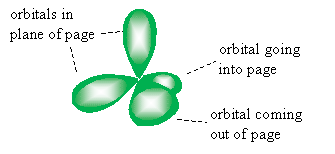A single carbon-carbon bond is the result of the overlap of one of these orbitals to form a sigma (σ) bond.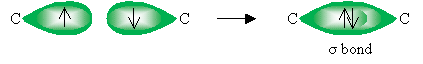So what about the case where carbon has a double (alkene) or triple (alkyne) bond? You may already see the difficulty, following the VSEPR model, of attempting to bend another orbital around the carbon atom to form a second σ bond-such would bring electrons from different orbitals close to each other, increasing the energy of the system owing to strong repulsive forces.

Consider the possibility, however, of the carbon atom only hybridizing three of its orbitals: the 2s orbital and two 2p orbitals. This would leave one 2p orbital, which has lobes above and below an imaginary "nodal" plane that passes through the carbon atom. The three hybridized orbitals-which we call sp2 hybrid orbitals-would then, according to the VSEPR model, all reside in a plane perpendicular to the unhybridized orbital. A sketch of these orbitals is shown below.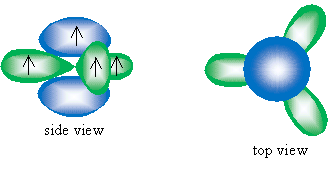Note that two carbon atoms could then approach and have the 2p orbital and one sp2 orbital overlap to form two bonds. This second bond, which corresponds to overlapping 2p orbitals, does not involve a linear arrangement like the σ bond. Instead, these orbitals are perpendicular to the line that intersects the two carbon atoms. This bond is called a pi (π) bond. A sketch of the overlapping orbitals that for a double (alkene) bond between two carbons is shown below. For clarity, only the relevant orbitals are shown; the drawing is not to scale.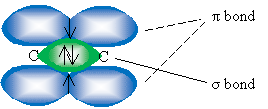For a triple bond, only two orbitals hybridize: the 2s orbital and one 2p orbital to form two sp hybrid orbitals. The sketch below shows these orbitals: the green orbital is the sp orbital, and the blue and red orbitals are the remaining unhybridized 2p orbitals.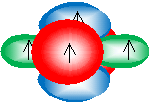Instead of one π bond, a triple bond between two carbon atoms involves two π bonds in perpendicular planes (the blue and red 2p orbitals shown above overlap to form two π bonds). One of the hybridized orbitals still forms a σ bond, for a total of three bonds between the two carbon atoms. Although the details may vary somewhat, this model can also help us visualize and understand multiple bonds involving atoms other than carbon.

The Three-Dimensional Structure of Alkenes and Alkynes

The study of molecules in three dimensions, or stereochemistry. But to properly understand the structure of alkanes and alkynes, and the properties of these molecules, we must consider how their bonding characteristics affect their behavior. Note that in a single carbon-carbon bond-that is, a σ bond-the structure is symmetric about the imaginary line between the two carbon atoms.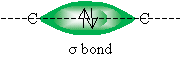Thus, we could rotate one carbon atom about this line (or axis) without affecting the shape and character of the bond. But in the case of a double bond, the nature of the π bond prevents this kind of rotation of a single carbon atom, short of causing strain on the molecule and increasing its associated energy. If we look at the situation somewhat simplistically, we can imagine that rotating one carbon atom would "twist" the π bond, causing it to break.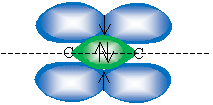Thus, an alkenyl group (an alkyl group that includes a double bond) forms a rigid 'X' shape.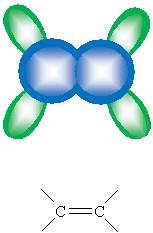Because of this rigid structure, the following molecules are actually different, even though according to our rules of nomenclature thus far, they are identical.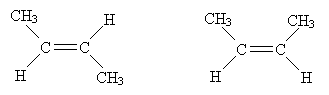Note that a methyl group (CH3) is spatially larger than a proton (H); thus, the molecule on the right is more "crowded" than the molecule on the left. Our VSEPR model can actually help us in this regard: because electron orbitals seek to be as far from each other as possible, the molecule on the left is the lower-energy (and thus preferred) form of 2-butene. This crowding of the molecule on the right causes van der Waals strain. But we must amend our nomenclature slightly to account for the differences between these forms. We use the prefix trans- to represent the case on the left and cis- to represent the case on the right. Molecules that differ in their three-dimensional forms are called stereoisomers.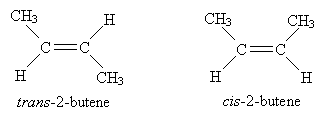In the case of alkynes, the two π bonds also form a rigid structure, but the VSEPR model indicates that the remaining bond for each carbon is antiparallel with the carbon-carbon triple bond. Thus, for instance, acetylene is a linear molecule (shown below), as is 2-butyne.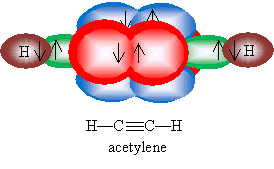Alkenes: Elimination Reactions

At this point, our focus shifts more toward chemical reactions and away from the physics of molecular bonding and structure. These physics concepts, however, will guide our study of chemical reactions. We turn, then, to how alkenes and alkynes can be formed from alkanes and other simple molecules. An elimination reaction, as the name indicates, involves removing some portion of a molecule to form a new structure. The general form of this reaction is shown below, where X and Y are substituent groups of the alkane. The unattached bonds above and below the alkane and alkene are left unspecified.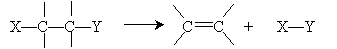We will only study 1,2 eliminations-also known as beta (β) eliminations. These names come from the convention of identifying the two carbon atoms in the molecule above as carbons 1 and 2 (or α and β).

One type of β elimination reaction is dehydrogenation. In this reaction, an alkane subjected to high temperatures (750° Celsius) expels hydrogen gas to form an alkene. The example of propane forming propene is illustrated below.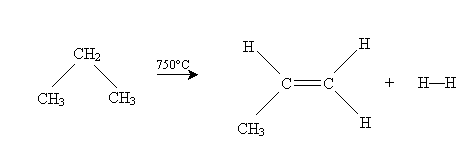A more involved process, but one that can be achieved at lower temperatures, is dehydration of alcohols. When an alcohol is immersed in a strongly acidic solution, alkenes can form by one of two processes (which attempt to describe the chemical process): the E1 mechanism or the E2 mechanism. Consider ethanol in a solution of sulfuric acid (H2SO4) at a temperature of about 160°C. According to the Br nsted-Lowry model, sulfuric acid produces hydronium ions (H3O+), which interact with the hydroxyl group of ethanol.This step occurs at a relatively fast rate. The next step, which occurs more slowly and is therefore the rate-determining step for the overall dehydration reaction, differentiates the E1 and E2 mechanisms. For the E1 mechanism (elimination involving one molecule), a carbocation (molecule with a positively charged carbon atom) is formed.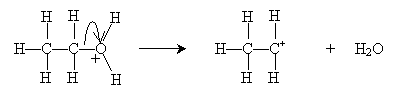The carbocation is a strong acid that readily reacts with water (a relative base) to form ethene, in this case.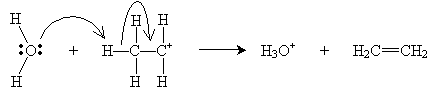The E2 mechanism combines these two steps, meaning two molecules are involved in the elimination. This step obviates the need for the highly reactive carbocation stage.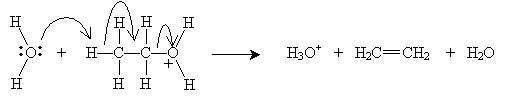Note that the presence of the acid catalyzes elimination of a proton (H) and a hydroxyl group (OH), which form water. The hydronium ions (H3O+) remain essentially unchanged by the reaction. The overall reaction, then, is the following, where the reaction takes place at about 160°C in the presence of, for example, sulfuric acid (H2SO4):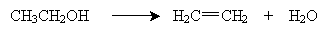But what about cases like 2-pentanol? Where will the double bond form?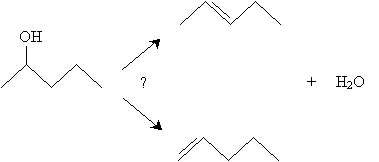According to Zaitsev's rule, a β elimination reaction (such as the dehydration reaction above) will predominantly yield the alkene that is most highly substituted. Note that for 2-pentanol, 2-pentene has a more highly substituted double bond than 1-pentene; thus, 2-pentene is the preferred product. Furthermore, as we noted previously, cis stereoisomers are less preferred forms than trans stereoisomers. As a result, we can expect the dehydration of 2-pentanol to yield mostly trans-2-pentene.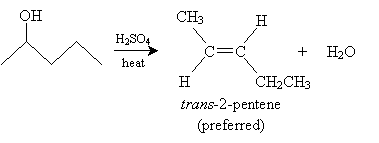Practice Problem: Draw the E2 mechanism for dehydrogenation of 2-methylcyclobutan-1-ol in sulfuric acid.

Solution: First, draw the molecule of interest: 2-methylcyclobutan-1-ol.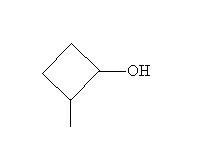The first step of the mechanism is interaction with a hydronium ion (H3O+) as follows.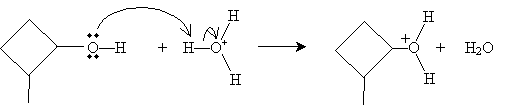The E2 step involves reaction with water to form a cycloalkene. Applying Zaitsev's rule, we know that the preferred result is a double bond that forms the most highly substituted alkene-in this case, the double bond forms with the methyl-substituted carbon.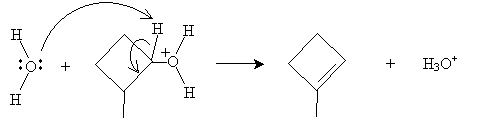The preferred product of the reaction is thus 1-methylcyclobutene.

Another reaction yielding alkenes is dehydrohalogenation of alkyl halides. In this case, an alkyl halide eliminates a hydrogen halide molecule to form an alkene, as summarized below.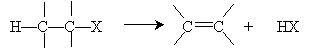Dehydrohalogenation can be performed in the presence of a base, following either an E1 or E2 mechanism. For the E2 reaction (two molecules are involved in the elimination) involving chloroethane in the presence of sodium methoxide (NaOCH3, which ionizes to form Na+ and OCH3- ions), the following process occurs: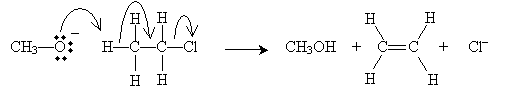The E1 reaction (the elimination involves one molecule) in this case is the following. Here, we illustrate dehydrohalogenation of chloroethane in ethanol. The first step (the rate-limiting step) involves dissociation of a halide ion: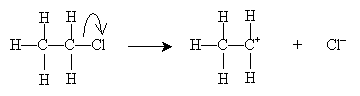The highly reactive carbocation then undergoes an acid-base reaction with ethanol.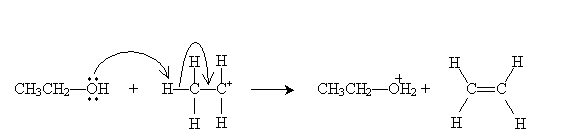Practice Problem: Draw the E1 dehydrohalogenation reaction of cyclohexyl chloride in ethanol.

Solution: The reaction starts with the elimination of a chloride ion, creating a cyclohexyl carbocation.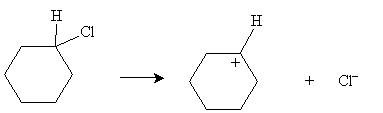The next step is reaction of the carbocation (which acts as a Lewis acid) with ethanol (which acts as a Lewis base) to form cyclohexene.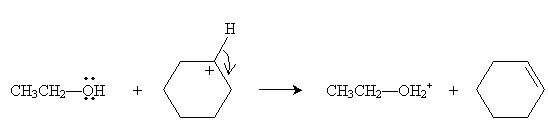You may notice that the reaction doesn't explicitly produce HCl as indicated by the overall reaction at the beginning of the discussion of dehydrohalogenation. Note, however, that HCl is a strong acid that tends to dissociate into protons and chloride ions in solution: our reaction produces chloride ions, and ethanol "captures" protons, forming a positively charged conjugate acid.

Alkynes: Dehydrogenation

Although we will not spend much time covering synthesis of alkynes, we can note that alkynes can be formed from alkenes by the same process of dehydrogenation that creates alkenes from alkanes. In the example shown below, heat added to ethene (ethylene) yields acetylene (an alkyne) and hydrogen gas.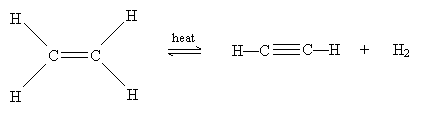Explore